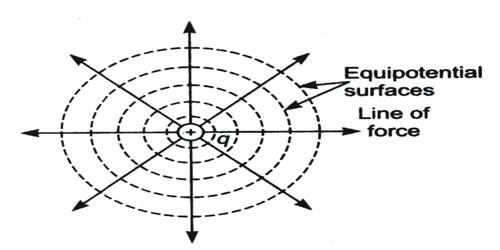# Explanation of Equipotential Surface

Explanation of Equipotential surface

Any surface over which the potential is constant is called an equipotential surface. In other words, the potential difference between any two points on an equipotential surface is zero.

Explanation: The equation of electric potential at a distance ‘r’ from the point charge + q is,

V = (1/4πε0) (q/r)Here q and ε0 are constants. So, the potential at a distance ‘r’ in all directions from the point charge will be same. In three dimensions surface of distance ‘r’ is a spherical surface. All points on this spherical surface will have equal potential; so it is an equipotential surface. For different values of r, we can have innumerable spheres of equal potential surfaces. In the figure, equipotential surfaces in two dimensions have been shown by drawing circles.

As the distance from the point charge increases the value of potential decreases.

Since all points on an equipotential surface have the same potential, so potential difference between any two points on that surface will be zero. Hence, work done will be zero as the potential difference is zero. So, in transferring a charge from one point to another on an equipotential surface, it does not need to do any work. The electric field intensity at any point on an equipotential surface acts perpendicular to that surface at that point.

Work Example: What will be the work done if a unit positive charge is transferred from one point to another point in an equipotential surface?

Explain. Potential of any two points in the equipotential surface is same. So, the potential difference between those two points will be Zero. According to the definition of potential difference work done in transferring a unit positive charge from a point to another point is equal to the potential difference between these two points. As the potential difference between any two points in the equipotential surface is zero, so work is done in transferring a unit positive charge from one point to another point will be zero.